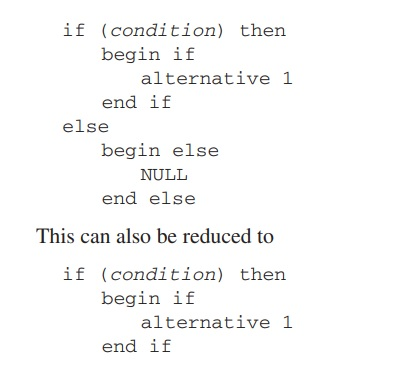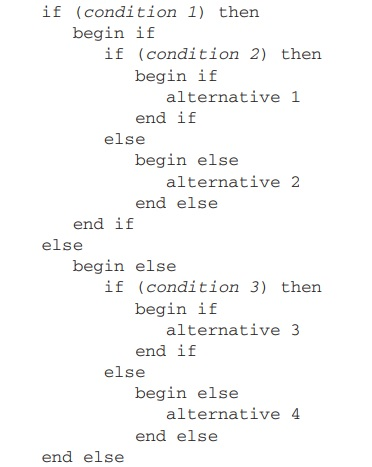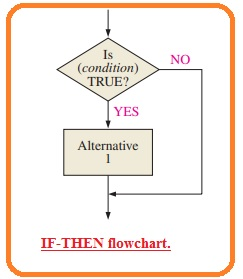Hello, friends welcome to another post in this post we will Discuss concept of conditional execution. The basic sequential program is restricted to some point since it supposes that the conditions for the program will always constant and will not vary from the certain process flow. The conditional execution rises the power and benefits of programs.

Conditional execution permits the program to do testing for certain situations and finds instruction if to be implemented. So let’s get started with Discuss the concept of conditional execution.

#### Discuss concept of conditional execution

• The flowchart which denotes the conditional execution apply the decision block. The decision block denotes the testing situations and series of commands that the program has to follow based on the outcomes of test.
• Program results finally always have 2 certain results therefore programs should use testing and outcomes which will always produce true or false or yes or no.
• For instance, the program cannot straightly apply the test like what this temperature. the program should find the temperature and these tests find parameters contrary to certain parameters.
• Programs uses 2 main category of conditional results. the IF THEN ELSE command and the case instruction.

#### IF-THEN-ELSE Instruction or Command

• IF THEN ELSE command applies the mostly elementary shape of conditional execution.
• If the outcomes of the test is TRUE then the program will apply one set of commands. If outcomes are FALSE that the program will implement an alternate group of commands.
• The simple pseudocode for IF THEN ELSE command is given here.
• The special shape of the IF THEN ELSE command is the IF-THEN command for that the ELSE part of the command does nothing.
• THe simple pseudocode for IF THEN is shown in the below figure.• As the IF THEN ELSE command looks to be restricted since it provides 2 ways one or either these two wasy can be IF THEN ELSE command.
• By similar category of commands for the miny parts of commands is known as nesting. The pseudocode for certain categories of nested IF THEN ELSE command is given here.#### CASE Instruction

• The CASE command is a certain category of nested IF THEN ELSE command which do comparison the program parameters contrary to the set of certain parameters to choose the code of the process.
• The flowchart is shown in below figure.• The above figure indicates the operation of CASE commands. Observe that the instruction for any case or default process do nothing.
• The CASE command finds that or not variable equal to certain parameters than whether the situation is TRUE or FALSE as in IF THEN ELSE conditions.
• IF THEN ELSE commands test larger than and less than equal or not equal to the situation.
• We can use IF THEN ELSE command to control these restrictions with the CASE commands.

That is a detailed post about the concept of conditional execution if you have any further queries ask in the comments. Thanks for reading.